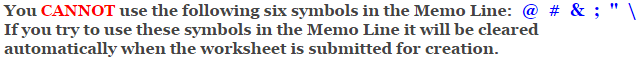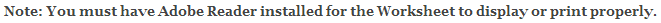Number Bonds Worksheets

Number Bonds Trees Worksheets

This Number Bonds Trees Worksheet is great for testing children in their ability to solve number bonds problems for a given sum in a tree format. A number bond is a mental picture of the relationship between a number and the parts that combine to make it. The concept of number bonds is very basic, an important foundation for understanding how numbers work. A whole thing is made up of parts. If you know the parts, you can put them together (add) to find the whole. If you know the whole and one of the parts, you take away the part you know (subtract) to find the other part.

Number bonds let children see the inverse relationship between addition and subtraction. Subtraction is not a totally different thing from addition; they are mirror images. To subtract means to figure out how much more you would have to add to get the whole thing.

 For example: ___ + 7 = 15 ___ + 9 = 15

This worksheet has the problems arranged in the form of a tree.

For number bond problems written as horizontal missing number problems, use the Number Bonds Worksheets

This worksheet will generate 12 problems per page. You may select from 5 to 99 for the sum to be used in the problems.

If you select a sum that is less than 12 some of the problems on the worksheet will repeat.

Language for the Number Bonds Worksheet

 English German Albanian Spanish Swedish Italian French Turkish Polish Norwegian

Memo Line for the Number Bonds Worksheet

You may enter a message or special instruction that will appear on the bottom left corner of the Number Bonds Worksheet.Number Bonds Worksheet Answer Page

Now you are ready to create your Number Bonds Worksheet by pressing the Create Button.

 Recommended Videos

If You Experience Display Problems with Your Math WorksheetClick here for More Number Bonds Worksheets